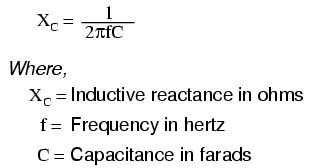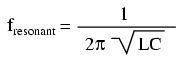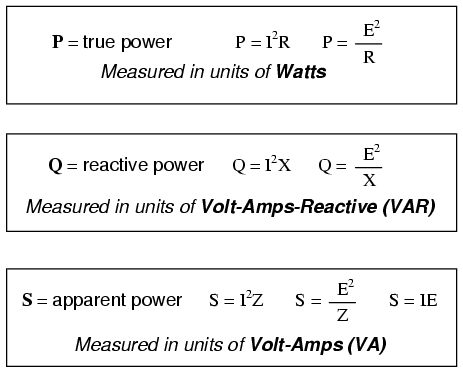# 1.08: AC Circuit Equations

## Inductive reactance## Capacitive reactanceZL = R + jXL

ZC = R - jXC

## Ohm’s Law for AC## Series and Parallel ImpedancesNOTE: All impedances must be calculated in complex number form for these equations to work.

## ResonanceNOTE: This equation applies to a non-resistive LC circuit. In circuits containing resistance as well as inductance and capacitance, this equation applies only to series configurations and to parallel configurations where R is very small.

## AC power